## Intermediate Python for Data Science: Looping Data StructureAfter the matplotlib for visualization, introduction to dictionaries and Pandas DataFrame, follows by logical, Boolean and comparison operators with if-elif-else control flow and now, comes to the last part, the while loop, for loop and loop for a different data structure.

In Python, some of the objects are iterable which means it loops through the object in a list, for example, to get each element. It loops through a string to capture each character in the string. A for loop iterates over a collection of things and while loop can do any kind of iteration within the block of codes, while some condition remains True

## For Loop

The main keywords are for and in. It uses along with colon (:) and indentation (whitespace). Below is the syntax,

```#loop statement
my_iterable = [1,2,3]
for item_name in my_iterable:
print(item_name)
```

I used two iterator variables (index, area) with enumerate(), for example, the sample code below. enumerate() loops over something and has an automatic counter, then returns an enumerate object.

```# areas list
areas = [11.25, 18.0, 20.0, 10.75, 9.50]

# Change for loop to use enumerate() and update print()
for index, area in enumerate(areas) :
print("room " + str(index) + ": " + str(area))

#Output:
"""
room 0: 11.25
room 1: 18.0
room 2: 20.0
room 3: 10.75
room 4: 9.5
"""
```

Another example utilizes a loop that goes through each sublist of house and prints out the x is y sqm, where x is the name of the room and y is the area of the room.

```# house list of lists
house = [["hallway", 11.25],
["kitchen", 18.0],
["living room", 20.0],
["bedroom", 10.75],
["bathroom", 9.50]]

# Build a for loop from scratch
for x in house:
print("the " + str(x) + " is " + str(x) + " sqm")

# Output:
"""
the hallway is 11.25 sqm
the kitchen is 18.0 sqm
the living room is 20.0 sqm
the bedroom is 10.75 sqm
the bathroom is 9.5 sqm
"""
```

Definition of enumerate() can be found here. My post on for loop is here.

## While Loop

The main keyword is while, colon (:) and indentation (whitespace). Below is the syntax,

```# while loop statement
while some_boolean_condition:
# do something

# Examples
x = 0
while x < 5:
print(f'The number is {x}')
x += 1
```

An example of putting an if-else statement inside a while loop.

```# Initialize offset
offset = -6

# Code the while loop
while offset != 0 :
print("correcting...")
if offset > 0:
offset = offset - 1
else:
offset = offset + 1
print(offset)

# Output:
"""
correcting...
-5
correcting...
-4
correcting...
-3
correcting...
-2
correcting...
-1
correcting...
0
"""
```

My post on while loop is here.

## Loop Data Structure

Dictionary:
If you want to iterate over key-value pairs in a dictionary, use the items() method on the dictionary to define the sequence in the loop.

```for key, value in my_dic.items() :
```

Numpy Array:
If you want to iterate all elements in a Numpy array, use the nditer() function to specify the sequence.

```for val in np.nditer(my_array) :
```

Some examples as below:

```# Definition of dictionary
'norway':'oslo', 'italy':'rome', 'poland':'warsaw', 'austria':'vienna' }

# Iterate over europe
for key, value in europe.items():
print("the capital of " + key + " is " + value)

# Output:
the capital of austria is vienna
the capital of norway is oslo
the capital of italy is rome
the capital of spain is madrid
the capital of germany is berlin
the capital of poland is warsaw
the capital of france is paris
"""

# Import numpy as np
import numpy as np

# For loop over np_height
for x in np_height:
print(str(x) + " inches")

# For loop over np_baseball
for x in np.nditer(np_baseball):
print(x)

```

Loop over DataFrame explanation and example can be found in my post here.

## Day 39: Using Iterator for Big Data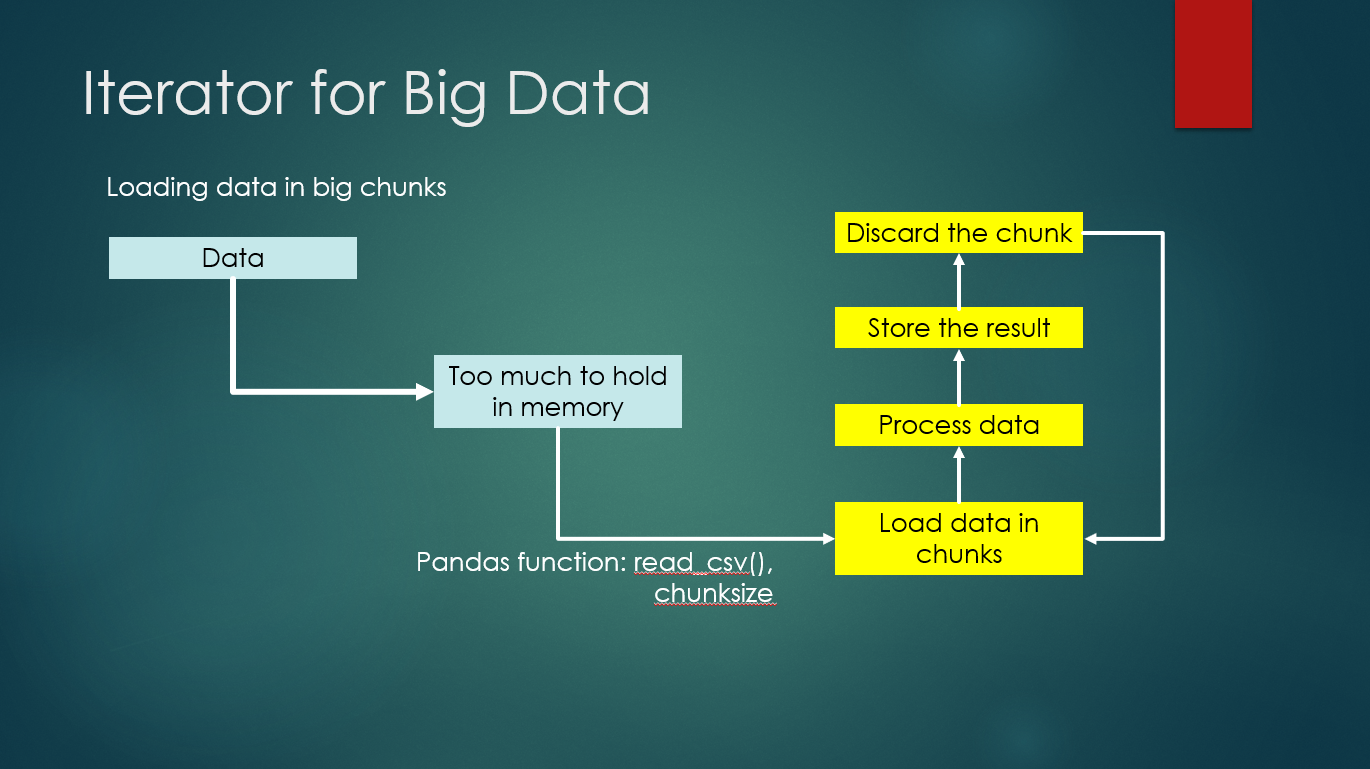The above illustrate the real scenario of data science where often they need to load a big chunk of data and sometimes it is too huge to be handled by the memory. The usage of Pandas, read_csv() function and setting the chunksize, it helps to load data in a smaller chunk, process the data and store the result somewhere before discard the chunk to load the next set to be processed. This is where iterator becomes useful.

Examples as below: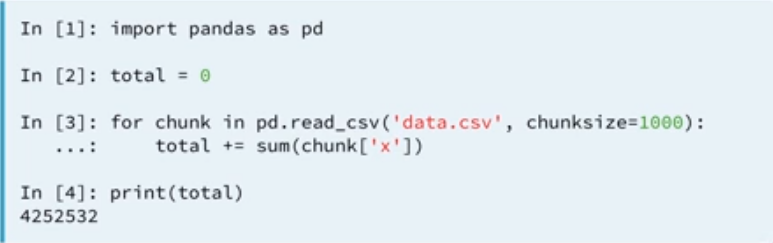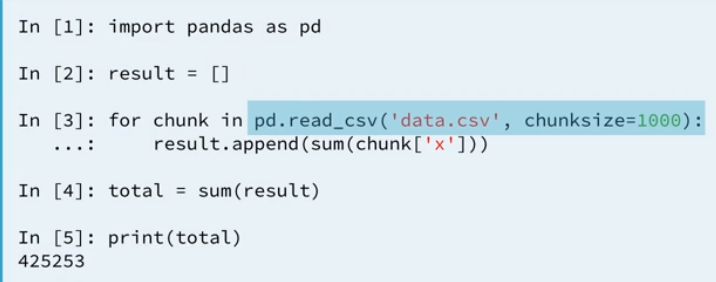Either we use a variable “total” to hold the sum’s result or we can create an empty dictionary to perform the same computation and it gives the same result. Below is the exercise I did in DataCamp’s online learning website using the Twitter’s data.

```# Initialize an empty dictionary: counts_dict
counts_dict = {}

# Iterate over the file chunk by chunk

# Iterate over the column in DataFrame
for entry in chunk['lang']:
if entry in counts_dict.keys():
counts_dict[entry] += 1
else:
counts_dict[entry] = 1

# Print the populated dictionary
print(counts_dict)
```

Create an empty dictionary, iterate over the csv file with chunksize is 10. Read the ‘lang’ column in the chunk and iterate again to get the count of each ‘lang’ in the .csv file. The output on the screen when executed is:

{‘en’: 97, ‘et’: 1, ‘und’: 2}

Let us convert the above code into an user defined function and takes three parameters, the csv filename, the chunk size and column name in the csv file. The updated version of the code with user defined function looks as below:

```# Define count_entries()
def count_entries(csv_file, c_size, colname):
"""Return a dictionary with counts of
occurrences as value for each key."""

# Initialize an empty dictionary: counts_dict
counts_dict = {}

# Iterate over the file chunk by chunk

# Iterate over the column in DataFrame
for entry in chunk[colname]:
if entry in counts_dict.keys():
counts_dict[entry] += 1
else:
counts_dict[entry] = 1

# Return counts_dict
return counts_dict

# Call count_entries(): result_counts
result_counts = count_entries('tweets.csv', 10, 'lang')

# Print result_counts
print(result_counts)
```

It gives the same result as the previous code.

Summary of the day:

• Iterator for Big Data.
• Using dictionaries, for loop statement to iterate data.
• Create an user defined function for the above and call to the function to print out result.

## Day 34: Using Pandas with DictionaryBelow is an exercise I did in DataCamp whereby, it required to use pandas package and read/import a .csv file into a DataFrame. Then, with a dictionary called ‘langs_count’, and a for loop iteration over the column named ‘lang’ in the DataFrame, to perform some actions.

Loop through each entry in the DataFrame, if the ‘lang’ is not found in the dictionary, then add the ‘lang’ with default value 1 into the dictionary, else add the current value with 1.

At first, it sounds a bit confused to me. Let us go through the codes and get the above statements understood. My code snippet is as below:

```# Import pandas
import pandas as pd

# Import Twitter data as DataFrame: df

# Initialize an empty dictionary: langs_count
langs_count = {}

# Extract column from DataFrame: col
col = df['lang']

# Iterate over lang column in DataFrame
for entry in col:

# If the language is in langs_count, add 1
if entry in langs_count.keys():
langs_count[entry] =  langs_count[entry] + 1
# Else add the language to langs_count, set the value to 1
else:
langs_count[entry] = 1

# Print the populated dictionary
print(langs_count)
```

As mentioned earlier, it imports the Pandas packages and it reads the .csv file using pandas’ read_csv() function. Next line, it creates an empty dictionary calls ‘langs_count’.

Up to here, it is straightforward.

Next line, it extracts the column ‘lang’ from the DataFrame and stores it in a variable calls ‘col’. You can do a print to check what is in df and col respectively to check the content inside.

Then, using a for loop to iterate the entry found in col. In other words, iterates each lang column in the DataFrame. Inside a for loop, we have an if-else condition check.

In dictionary, .key() returns a view object of all the keys in a dictionary.

The condition checks, if the language is inside ‘lang_count’ dictionary, then add 1 (+1) to its current the value (dictionary has key and value as a pair) else, create a new language in ‘langs_count’ and set as value 1 (=1).

We can access the value by specifying the key,
langs_count[entry]

where entry is the key and the code returns the value. And, with that value add 1 (+1), it gets a new value. Example like the price_lookup[‘apple’], it works the same.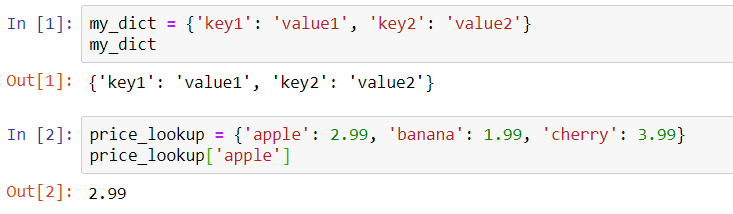Otherwise, we need to create a new item inside the dictionary by defining its key and value with this code,
langs_count[entry] = 1

This is how we add a new item, the same method used in the below example,
d[‘k3’] = 300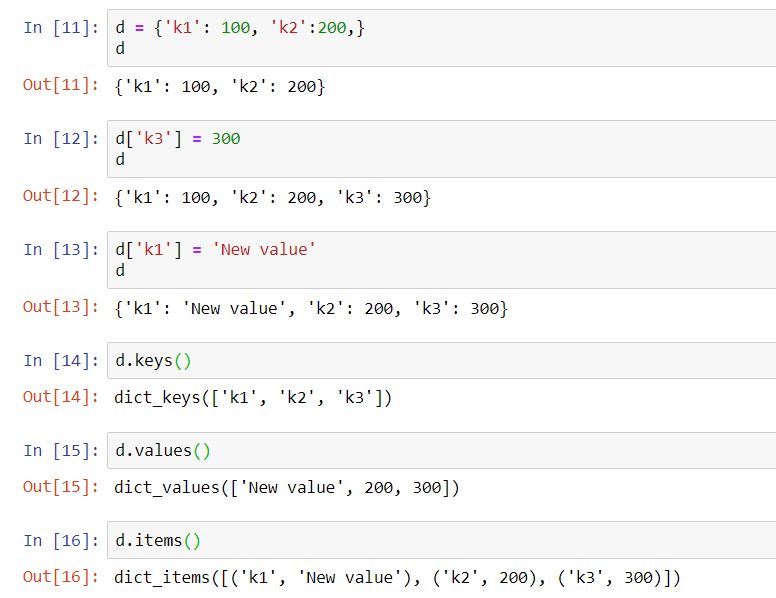Lastly, when the print(langs_count) is called, it prints out the dictionary as below:

```print(langs_count)
{'en': 97, 'und': 2, 'et': 1}

<script.py> output:
{'en': 97, 'und': 2, 'et': 1}
```

Based on the codes, it tells us there are 97 times of language ‘EN’ found in the dictionary and language ‘ET’ has 1 and I think undefined, ‘UND’ has 2.

The above codes can be converted into a user-defined function. By accepting two parameters with a return value statement, calls the function and stores the return value into a variable which we can print out the values. The codes as below:

```# Define count_entries()
def count_entries(df, col_name):
"""Return a dictionary with counts of
occurrences as value for each key."""

# Initialize an empty dictionary: langs_count
langs_count = {}

# Extract column from DataFrame: col
col = df[col_name]

# Iterate over lang column in DataFrame
for entry in col:

# If the language is in langs_count, add 1
if entry in langs_count.keys():
langs_count[entry] = langs_count[entry] + 1
# Else add the language to langs_count, set the value to 1
else:
langs_count[entry] = 1

# Return the langs_count dictionary
return langs_count

# Call count_entries(): result
result = count_entries(tweets_df,'lang')

# Print the result
print(result)
```

The result is the same as the previous codes. The difference is now, we can call using a function and substitute arguments required into the two parameters.

This is a basic data science function. There is more to learn in the upcoming topics in DataCamp, including error-handling functions.

Summary of the day:

• Using dictionary to store values.
• Using For loop and If-else conditions to workaround.

## Day 16: Python Control Flow using For LoopsIn Python, some of the objects are iterable which means I can loop through the object in a list for example, to get each element or I can loop through a string to capture each character in the string. A for loop can only iterate (loop) over a collections of things and while loop can do any kind of iteration as long as the condition is met.

The main keywords are for and in. It uses along with colon (:) and indentation (whitespace). Following is the syntax,

```#loop statement
my_iterable = [1,2,3]
for item_name in my_iterable:
print(item_name)
```

my_iterable is a list of number and the keyword “for” begins the for loop, with a given variable name called item_name. So, this for loop has a name called, item_name to represent the elements inside the list later. Next, the second keyword is “in” means it loops inside the variable called my_iterable. And, it follows by colon (:) to tell the program to execute everything after the colon. In this example, it prints the variable, item_name’s values one by one until end of the list.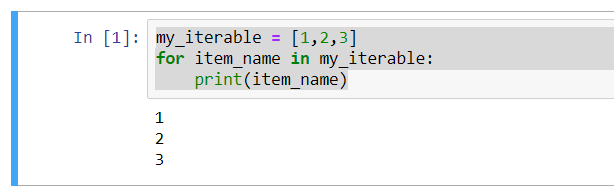Using the same list, you can also print something else instead of the elements of the variable, my_iterable. Example,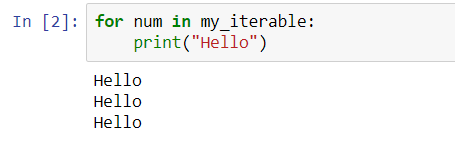It works too as the variable, my_iterable works as a counter and prints “Hello” thrice. Then, actually I do not need to have the variable named, num right? Yes, I can remove it with using underscore (_).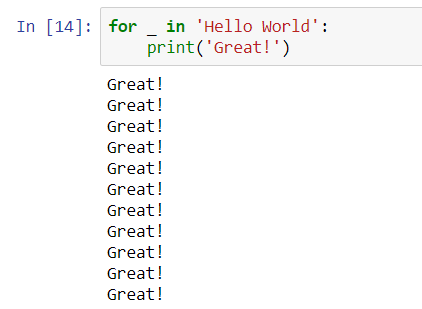In Python, when we do not intend to use the variable name anywhere, we can just put “_“. It works pretty cool.

It is simple to use and it can use with if statement I just shared in previous blog. Look into below example.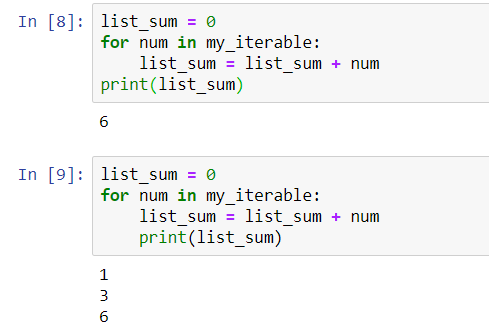When I place the print outside of the for statement (refer to ), print() is aligned with for keyword, means it prints the final value of the list_num after the loop ends. However, if I indent the print statement to be aligned with list_num’s sum operation, it prints each sum value of the list_sum until end of the list.

It depends on how do you want to display the information, the indentation of print statement positioning plays a role. If I put the print statement before the for statement, it prints 0 first and follows by the sum values of list_num in each iteration. Just give a try to confirm it 🙂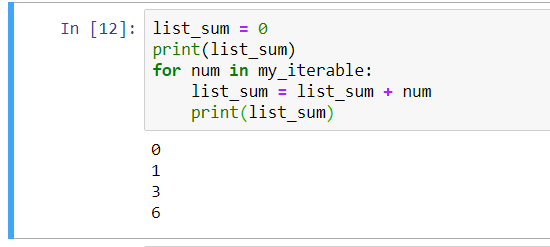Do you get the same output as me?

It works for string too as I mentioned earlier, example if I want to get each character in a string.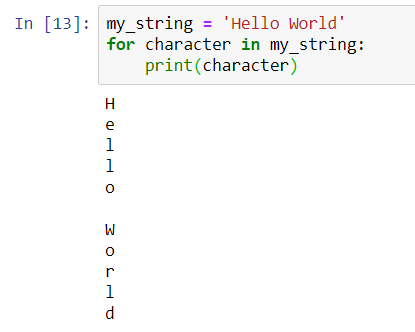Another example which I learned from online learning website is using tuple. Still remember tuple element is inside a parenthesis ()? Below examples are showing how to use tuple and tuple in a list in for statement.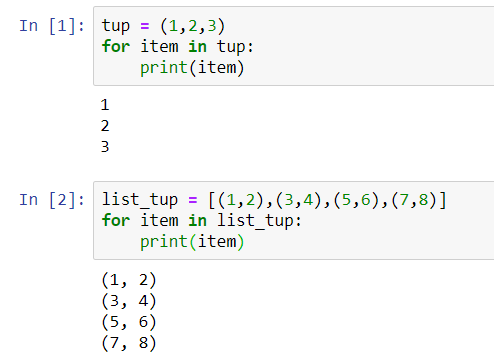In , it uses tuple and prints out the value one by one.
In  it uses a list of tuple, in a pair of two and it prints out 4 pairs of tuples.

Here is a jargon to learn in Python, tuple unpacking. It means unpack or extract the values into variable. The next codes shows how to do it. It creates variables in same structure as the tuple and print each variable separately. With this method, it can access each value individually instead. If want to just print variable a, then omit the print(b).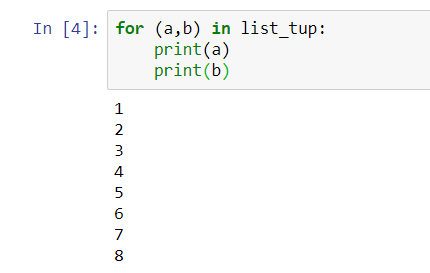Lastly, we can iterate through a dictionary, another type of collections in Python. Dictionary is unordered mappings, so in a large dataset, we may not able to get the values listed nicely and how to access the dictionary key and value will help.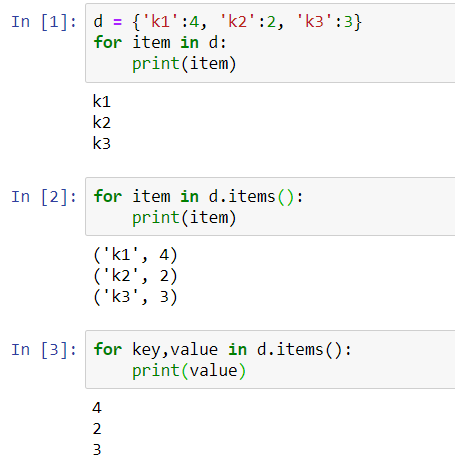By default, using for statement in a dictionary, it returns the “key”, that is shown in the  example. To access the key-value pair, it uses d.items(), which is shown in the  example. And, with this structure, I am sure you know, we can access each element if we use key,value similarly how we try to access tuple earlier.

Fantastic!

Now, I start to think back the time when I looked into the Scala codes, it did has similar things. I am sorry, I am supposed to be a developer who knows how to code Scala but I did not really learn it well. Alright, I am going to stop here and hopefully, if there is more interesting loop examples to be shared in the future, I will update it.

Summary of the day:

• Control flow using For loops.
• Important keywords: for, in.
• The magic using underscore (_).
• Tuple unpacking. (Quite interesting topic to look at).